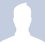Circle inscribed in a triangle with triangle inscribed circle...

An equilateral triangle is inscribed in a circle, which (the circle) is also inscribed in another equilateral triangle. what is the ratio of the areas of the inner triangle and outside triangle?Note by Alan Liang
6 years, 8 months ago

This discussion board is a place to discuss our Daily Challenges and the math and science related to those challenges. Explanations are more than just a solution — they should explain the steps and thinking strategies that you used to obtain the solution. Comments should further the discussion of math and science.

When posting on Brilliant:

• Use the emojis to react to an explanation, whether you're congratulating a job well done , or just really confused .
• Ask specific questions about the challenge or the steps in somebody's explanation. Well-posed questions can add a lot to the discussion, but posting "I don't understand!" doesn't help anyone.
• Try to contribute something new to the discussion, whether it is an extension, generalization or other idea related to the challenge.
• Stay on topic — we're all here to learn more about math and science, not to hear about your favorite get-rich-quick scheme or current world events.

MarkdownAppears as
*italics* or _italics_ italics
**bold** or __bold__ bold

- bulleted
- list

• bulleted
• list

1. numbered
2. list

1. numbered
2. list
Note: you must add a full line of space before and after lists for them to show up correctly
paragraph 1

paragraph 2

paragraph 1

paragraph 2

> This is a quote
This is a quote
# I indented these lines
# 4 spaces, and now they show
# up as a code block.

print "hello world"
# I indented these lines
# 4 spaces, and now they show
# up as a code block.

print "hello world"
MathAppears as
Remember to wrap math in $$...$$ or $...$ to ensure proper formatting.
2 \times 3 $2 \times 3$
2^{34} $2^{34}$
a_{i-1} $a_{i-1}$
\frac{2}{3} $\frac{2}{3}$
\sqrt{2} $\sqrt{2}$
\sum_{i=1}^3 $\sum_{i=1}^3$
\sin \theta $\sin \theta$
\boxed{123} $\boxed{123}$

Sort by:

according to me it should be 1/4

- 6 years, 7 months ago

$\frac{1}{4}$

- 6 years, 8 months ago

it can be done by rotation, rotate the inner triangle by 60 degrees with centroid fixed, so now the inner triangle has its vertices on the midpoints of the smaller triangle!! so 1/4 is tthe answer.

- 6 years, 8 months ago

1/4

- 6 years, 8 months ago

1/4

- 6 years, 8 months ago

Yeah.... I'll go with 1:4

- 6 years, 8 months ago

It's 1/4.

- 6 years, 8 months ago

The ratio is $1/4$. Simply rotate the smaller triangle so that its corners lie at the midpoints of the sides of the larger triangle.

- 6 years, 8 months ago

i like yr answer

- 6 years, 8 months ago

\frac{1}{4}

- 6 years, 8 months ago

1/5

- 6 years, 8 months ago

Two triangles are similar, altitudes are 3R and 3R/2 => ratio of altitudes = 1 : 2

=> ratio of areas = 1 : 4

- 6 years, 8 months ago

i think the ratio is 1/4

- 6 years, 8 months ago

1:3

- 6 years, 8 months ago

Fail.

- 6 years, 8 months ago

Rotate the inner triangle so that it is the medial triangle of the outside triangle.

- 6 years, 8 months ago

Refer here for the figure. Let $O$ be the center of the circle and the radius of the circle be $r$. The equilateral triangle $\Delta ABC$ is inscribed inside the circle and the circle is inscribed inside the equilateral triangle $\Delta DEF$. Let $AA'$ be perpendicular to $BC$ and $DD'$ be perpendicular to $EF$. Since $\Delta ABC$ and $\Delta DEF$ are equilateral triangle, $AA'$ and $DD'$ are both medians to the respective sides from respective vertices and both pass through $O$, which is also the centroid. Recall that the centroid divides the medians in the ratio $2:1$. Hence, we get that $\dfrac{AO}{AA'} = \dfrac{DO}{DD'} = \dfrac23 \implies AA' = \dfrac{3r}2; DD' = 3r$ Hence, $\dfrac{\text{Area of }\Delta ABC}{\text{Area of }\Delta DEF} = \dfrac{\dfrac12 \cdot AA' \cdot BC}{\dfrac12 \cdot DD' \cdot EF} = \dfrac{AA'}{DD'} \cdot \dfrac{BC}{EF} = \dfrac{AA'}{DD'} \cdot \dfrac{A'C}{D'F} = \left(\dfrac{AA'}{DD'} \right)^2 = \dfrac14$

- 6 years, 8 months ago

I think it's $\frac{1}{4}$...

or maybe I have made wrong in calculations...

- 6 years, 8 months ago

Thanks, but could you give me steps on how you did it?

- 6 years, 8 months ago

Don't you know that $r=\frac{A}{s}$ if the circle is inscribed in a triangle. With r=radius of circle, A=area of triangle and s=a half of triangle's perimeter... $r=\frac{abc}{4A}$ if the circle is circumcircle with a,b,c=side of the triangle. Or I'll give the link for you? Sorry I can't draw the picture for you.

- 6 years, 8 months ago